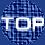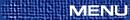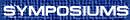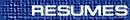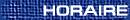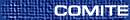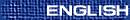Number Theory-in Honour of David Boyd / Théorie des nombres-en l'honneur de David Boyd
(Peter Borwein and Michael Bennett, Organizers)

JIM ARTHUR, Toronto
To be announced

IMIN CHEN, Simon Fraser University, British Columbia
On relations between induced representations for GL2(Z/p2Z)

We will exhibit a relation between induced representations for GL2(Z/p2Z) analogous to the one for GL2(Z/pZ) which relates the induced representations from split and non-split Cartan subgroups. Some possible applications to modular curves will be discussed. This work is joint with B. deSmit and M. Grabitz.

KWOK KWONG (STEPHEN) CHOI, Simon Fraser University, Burnaby, British Columbia  V5A 1S6
Small prime solutions of quadratic equations

In this talk, we will study the small prime solutions of certain diophantine equations by using the Hardy-Littlewood (circle) method. In particular, we prove the following. If b1,...,b5 are non-zero integers, then the quadratic equation b1p12+¼+b5p52=n has prime solutions satisfying pj << Ö[(|n|)]+max{|bj|}20+e. In contrast to the earlier works which treat the enlarged major arc by the Deruring-Heilbronn phenomenon about the Siegel zero. We will explain the possible existence of Siegel zero does not have special influence and hence the Deuring-Heilbronn phenomenon can be avoided. This observation enables us to get better results without numerical computations.

KARL DILCHER, Dalhousie University, Halifax, Nova Scotia
Bernoulli numbers and confluent hypergeometric functions

If the reciprocal of the confluent hypergeometric function M(s+1;r+s+2;z) is taken as an exponential generating function, the resulting sequences of numbers have many properties resembling those of the Bernoulli numbers, which arise when r=s=0. Other special cases include van der Pol numbers and generalized van der Pol numbers (for positive integers r=s), as well as various sequences of combinatorial numbers and linear recurrence sequences of arbitrary orders. Explicit expressions resembling Euler's formula involve sums of powers of the zeros of the corresponding confluent hypergeometric functions.

RON FERGUSON, Simon Fraser University, Burnaby, British Columbia
Units in integral cyclic group rings for order 4p

For a finite abelian group A, the group of units in the integral group ring Z A may be written as the direct product of its torsion units ±A with a free group U2A. Of finite index in U2A is the group WA, the elements of U2 which are mapped to cyclotomic units by each character of A. The order of U2A/WA depends on class numbers hd+ in real cyclotomic rings Z [zd+zd-1].

Of finite index in WA is the group of constructible units YA, for which a multiplicative basis may be explicitly written. The order of WA/YA is the circular index c(A). In many cases, for example where A is a p-group with p a regular prime, this index is trivial.

The theory is developed for determining c(Cn) where Cn is a cyclic group of order n=4p, for p prime, and finding a multiplicative basis for the group WCn. This is a continuation of the work of Hoechsmann for the case n=lp. The extension for a higher power, however, does require the introduction of new methods.

JOHN FRIEDLANDER, Toronto
Exponential sums over thin sets

We discuss results with H. Iwaniec and with I. Shparlinski on character and exponential sums over thin sets of integers.

KEVIN HARE, Simon Fraser University, British Columbia
Spectra of Pisot numbers

Here we study the spectrum that is the result of evaluating certain classes of polynomials at a value q > 1. Some classes of polynomials that have been looked at included those of height m, or those with coefficients restricted to ±. This talk with give a history of problems relating to these spectrums, the problems interrelationship with Pisot numbers, and some recent results in this area. Lastly this talk will describe some possible future directions for work in this area.

HERSHY KISILEVSKY, Concordia University, Montreal, Quebec
Vanishing and non-vanishing of twisted L-functions

Given an elliptic curve E over the rational field, Q, we compare the Mordell-Weil groups E(K) and E(Q) for cyclic cubic extensions K/Q. We expect that the rank E(K) is equal to the rank of E(Q) for almost all such K/Q, but that the rank actually increases for an infinite number of K. We describe our results on this problem, with particular reference to the rank 0 curve of conductor 37 which is unusually rich in this regard.

GREG MARTIN, University of Toronto, Toronto, Ontario  M5S 3G3
Squarefree values of trinomial discriminants

The discriminant of a trinomial of the form xn±xm±1 has the form ±nn ±(n-m)n-mmm if n and m are relatively prime. We investigate when these discriminants have nontrivial square factors. We explain various unlikely-seeming parametric families of square factors of these discriminant values: for example, when n º 2 mod 6 we have

 æè n2-n+13 öø 2 êê æè nn-(n-1)n-1 öø .
In addition, we discover many other square factors of these discriminants that do not fit into these parametric families. The set of primes whose squares can divide these sporadic values as n varies seems to be independent of m, and this set can be seen as a generalization of the Wieferich primes, those primes p such that 2p-1 º 1 mod p2. The truth about the density of squarefree values of these discriminants, and about the density of these ``sporadic'' primes, is still unclear. (Joint work with David Boyd)

KUMAR MURTY, Toronto
To be announced

RAM MURTY, Queen's University, Kingston, Ontario  K7L 3N6
Pair correlation conjecture and the Chebotarev density theorem

We will formulate a pair-correlation conjecture for the zeros of Artin L-functions analogous to a similar conjecture formulated by Montgomery in the theory of the Riemann zeta function. We will then investigate the consequences of such a conjecture for the error term in the effective version of the Chebotarev density theorem. (This is joint work with Kumar Murty.)

NATHAN NG, University of British Columbia, Vancouver, British Columbia
The summatory function of the Mobius function

We present some conditional results concerning the summatory function of the Mobius function, M(x). These results depend on a conjecture by Steve Gonek and Dennis Hejhal regarding negative discrete moments of the zeta function. The purpose of this talk is to show how this conjecture allows one to obtain much better results about M(x) than were previously known. Assuming the Riemann Hypothesis and the Gonek-Hejhal conjecture, we can show the weak Mertens conjecture is true and construct a limiting distribution attached to M(x). The constructed limiting distribution then reveals the behaviour of M(x). For example, we speculate that

 M(x)=W± æè x[1/2](logloglogx)[5/4] öø
based on properties of the constructed limiting distribution.

CHRISTOPHER PINNER, Kansas State University, Manhattan, Kansas  66506, USA
A bound for a complete exponential sum modp

We give an upper bound for the exponential sum åx=1p e(f(x)/p), p a prime and f a non-zero integer polynomial, of interest in cases where the Weil bound is worse than trivial. The result extends a technique of Konyagin for monomials to a general polynomial. Such bounds readily yield estimates for the corresponding polynomial Waring problem mod p; namely the smallest g such that f(x1)+¼+f(xg) º N mod p) is solvable in integers for any N.

This is joint work with Todd Cochrane.

DAMIEN ROY, University of Ottawa, Ottawa, Ontario
Interpolation on perturbations of cartesian products

In 1970, E. Bombieri and S. Lang used analytic results of P. Lelong to establish a Schwarz lemma for a well distributed set of points in Cn. Their result was extended to an interpolation lemma, first by D. W. Masser in the case of polynômials, then by M. Waldschmidt for analytic fonctions. J.-C. Moreau gave an analog of it over the reals and P. Robba in the p-adic realm. Robba also conjectured a p-adic interpolation lemma for the case where the set of points of interpolation is what he calls a perturbation of a product set, a situation which includes both the case of a well distributed set and the case of a cartesian product. In this talk, we present an algebraic proof of Robba's conjecture together with a generalization of it over the complex numbers.

CAM STEWART, University of Waterloo, Waterloo, Ontario
Multivariate Diophantine equations with many solutions

We shall discuss recent joint work with J.-H. Evertse, P. Moree and R. Tijdeman on S-unit equations and norm polynomial equations with many solutions.

MICHEL WALDSCHMIDT, Institut de Mathematiques de Jussieu
Polylogarithms and multiple zeta values

For a tuple s=(s1,¼,sk) of positive integers the power series

 Lis(z)= å n1 > ¼ > nk ³ 1 z1n1¼zknkn1s1¼nksk
defines an analytic function in the unit polydisk |zi| < 1 (1 £ i £ k). When s1 ³ 2 this function is continuous on the closed polydisk |zi| £ 1 (1 £ i £ k) and its value at the point z1=¼ = zk=1 is the multiple zeta value z(s). Several shuffle relations connect the different Lis and give rise to quadratic or linear algebraic dependence relations between the multiple zeta values. The main Diophantine conjecture is that all algebraic dependence relations between these numbers are consequences of these.

HUGH WILLIAMS, Department of Computer Science, University of Manitoba, Winnipeg, Manitoba  R3T 2N2
Some computational results concerning Yokoi's conjecture

Let d = m2+4 be the fundamental discriminant of the real quadratic field K = Q(Öd). Yokoi's Conjecture states that if the class number of K, written h(d), is 1, then m £ 17. It is known that there can be at most one exceptional value of m ( > 17) such that h(d) = 1, and that this exceptional value must exceed 10300000; it is also known that Yokoi's conjecture must hold if the extended Riemann hypothesis is true. In spite of this evidence for the truth of the conjecture, it seems that proving it is a very difficult problem, indeed.

In this paper we show how earlier arguments of Beck can be refined and implemented on a computer to restrict further those values of m which would produce a counter example to Yokoi's conjecture For example, we were able to use a computer to show that there are at most 36 possible residue classes for m (modulo 179) such that h(d) might be 1.

This is joint work with Jozsef Beck (Rutgers) and Edlyn Teske (Waterloo).